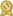Found 3800 trigonometry tutors – page 5 of 380.

Pembroke Pines 33028 · \$35/hour · teaches Statistics - Trigonometry

Dear Parents and Students: I have over 20 years of experience tutoring Math and Physics. I specialize in tutoring Algebra, Geometry, Trigonometry, Precalculus, Calculus, Statistics and Physics to high school...certified

Milford 03055 · \$40/hour · teaches Statistics - Trigonometry

Courses I have taught include algebra, trigonometry, precalculus, calculus, differential equations, statistics, discrete mathematics, and advanced engineering mathematics.certified

Huntersville 28078 · \$50/hour · teaches SAT Writing - Trigonometry

I tutor SAT, ACT, GRE , GMAT test prep, math 1-3, AFM, pre-calculus, high school calculus, prealgebra, algebra, trigonometry, geometry, German, and some other subjects. My Qualifications: I have been a full-time...certified

Hampton 30228 · \$50/hour · teaches Softball - Trigonometry

I formerly taught high school and middle school math (Pre-Algebra, Algebra I, Algebra II, Geometry, Pre-Calculus, and Trigonometry). I also taught all subjects to my 3 children (One graduated from college with...certified

Stamford 06902 · \$100/hour · teaches SAT Math - Trigonometry

This experience includes Algebra, Geometry, Algebra 2, Trigonometry, Precalculus and AP Calculus. I have been teaching in New York City for over 5 years and have experience working will all age levels.certified

Keller 76244 · \$50/hour · teaches SAT Math - Trigonometry

I am very passionate when it comes to math and science. My strongest strength is math. My favorite math is algebra, but I also have a deep understanding of statistic, geometry, trigonometry...certified

Long Beach 90803 · \$60/hour · teaches Statistics - Trigonometry

Hello! My name is David, and I hope to be the tutor you are looking for. I have almost 10 years of tutoring experience in all math subjects, including Algebra, Geometry, Trigonometry, Pre-Calculus, Calculus...certified

Saugerties 12477 · \$40/hour · teaches SAT Math - Trigonometry

I have taken several upper level math courses that include: Calculus 1-3, Introduction to Proofs, Axiomatic Geometry, Precalculus, Algebra 1-2, Trigonometry, Geometry, linear algebra, abstract algebra...certified

Olathe 66062 · \$30/hour · teaches SQL - Trigonometry

...trigonometry, precalculus and calculus. This current year of 2017-2018 I am teaching the following subjects: two high school students calculus, one high school student precalculus, one middle school student...certified

Bronx 10465 · \$125/hour · teaches SAT Math - Trigonometry

I have expertise in all levels of math from SAT, Integrated Algebra, Geometry, Trigonometry, Precalculus, Calc AB and BC, and college calculus. My rates are very affordable compared to local tutoring agencies...certified